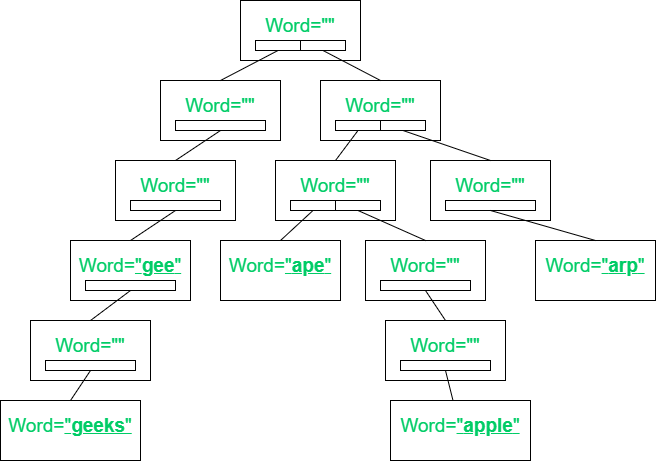Related Articles

# Spell Checker using Trie

• Difficulty Level : Hard
• Last Updated : 31 Mar, 2021

Given an array of strings str[] and a string key, the task is to check if the spelling of the key is correct or not. If found to be true, then print “YES”. Otherwise, print the suggested correct spellings.

Examples:

Input:str[] = { “gee”, “geeks”, “ape”, “apple”, “geeksforgeeks” }, key = “geek”
Output: geeks geeksforgeeks
Explanation:
The string “geek” not present in the array of strings.
Therefore, the suggested words are { “geeks”, “geeksforgeeks” }.

Input: str[] = { “gee”, “geeks”, “ape”, “apple”, “arp” }, key = “geeks”
Output: YES.Approach:The problem can be solved using Trie. The idea is to traverse the array of string, str[] and insert the string into the Trie such that each node of the Trie contains the character of the string and a boolean value to check if the character is the last character of the string or not. Follow the steps below to solve the problem:

• Initialize a Trie, say root, such that each node of the Trie consists of a character of a string and a boolean value to check if the character is the last character of the string or not.
• Traverse the array of strings arr[], and insert all the strings into the Trie.
• Finally, traverse the string key. For every ith character, check if the character is present in the Trie or not. If found to be true, then move to the next node of the Trie.
• Otherwise, print all possible strings whose prefix is the string key.

Below is the implementation of the above approach:

## C++

 `// C++ program to implement``// the above approach``#include ``using` `namespace` `std;` `// Structure of a Trie node``struct` `TrieNode {` `    ``// Store address of a character``    ``TrieNode* Trie;` `    ``// Check if the character is``    ``// last character of a string or not``    ``bool` `isEnd;` `    ``// Constructor function``    ``TrieNode()``    ``{` `        ``for` `(``int` `i = 0; i < 256; i++) {` `            ``Trie[i] = NULL;``        ``}``        ``isEnd = ``false``;``    ``}``};` `// Function to insert a string into Trie``void` `InsertTrie(TrieNode* root, string s)``{` `    ``TrieNode* temp = root;` `    ``// Traverse the string, s``    ``for` `(``int` `i = 0; i < s.length(); i++) {` `        ``if` `(temp->Trie[s[i]] == NULL) {` `            ``// Initialize a node``            ``temp->Trie[s[i]] = ``new` `TrieNode();``        ``}` `        ``// Update temp``        ``temp = temp->Trie[s[i]];``    ``}` `    ``// Mark the last character of``    ``// the string to true``    ``temp->isEnd = ``true``;``}` `// Function to print suggestions of the string``void` `printSuggestions(TrieNode* root, string res)``{` `    ``// If current character is``    ``// the last character of a string``    ``if` `(root->isEnd == ``true``) {` `        ``cout << res << ``" "``;``    ``}` `    ``// Iterate over all possible``    ``// characters of the string``    ``for` `(``int` `i = 0; i < 256; i++) {` `        ``// If current character``        ``// present in the Trie``        ``if` `(root->Trie[i] != NULL) {` `            ``// Insert current character``            ``// into Trie``            ``res.push_back(i);``            ``printSuggestions(root->Trie[i], res);``            ``res.pop_back();``        ``}``    ``}``}` `// Function to check if the string``// is present in Trie or not``bool` `checkPresent(TrieNode* root, string key)``{` `    ``// Traverse the string``    ``for` `(``int` `i = 0; i < key.length(); i++) {` `        ``// If current character not``        ``// present in the Trie``        ``if` `(root->Trie[key[i]] == NULL) {` `            ``printSuggestions(root, key.substr(0, i));` `            ``return` `false``;``        ``}` `        ``// Update root``        ``root = root->Trie[key[i]];``    ``}``    ``if` `(root->isEnd == ``true``) {` `        ``return` `true``;``    ``}``    ``printSuggestions(root, key);` `    ``return` `false``;``}` `// Driver Code``int` `main()``{` `    ``// Given array of strings``    ``vector str = { ``"gee"``, ``"geeks"``, ``"ape"``,``                           ``"apple"``, ``"geeksforgeeks"` `};` `    ``string key = ``"geek"``;` `    ``// Initialize a Trie``    ``TrieNode* root = ``new` `TrieNode();` `    ``// Insert strings to trie``    ``for` `(``int` `i = 0; i < str.size(); i++) {``        ``InsertTrie(root, str[i]);``    ``}` `    ``if` `(checkPresent(root, key)) {` `        ``cout << ``"YES"``;``    ``}``    ``return` `0;``}`

## Java

 `// Java program to implement``// the above approach``import` `java.io.*;` `// Structure of a Trie node``class` `TrieNode``{``    ` `    ``// Store address of a character``    ``TrieNode Trie[];` `    ``// Check if the character is``    ``// last character of a string or not``    ``boolean` `isEnd;` `    ``// Constructor function``    ``public` `TrieNode()``    ``{``        ``Trie = ``new` `TrieNode[``256``];``        ``for``(``int` `i = ``0``; i < ``256``; i++)``        ``{``            ``Trie[i] = ``null``;``        ``}``        ``isEnd = ``false``;``    ``}``}` `class` `GFG{` `// Function to insert a string into Trie``static` `void` `InsertTrie(TrieNode root, String s)``{``    ``TrieNode temp = root;` `    ``// Traverse the string, s``    ``for``(``int` `i = ``0``; i < s.length(); i++)``    ``{``        ``if` `(temp.Trie[s.charAt(i)] == ``null``)``        ``{``            ` `            ``// Initialize a node``            ``temp.Trie[s.charAt(i)] = ``new` `TrieNode();``        ``}` `        ``// Update temp``        ``temp = temp.Trie[s.charAt(i)];``    ``}` `    ``// Mark the last character of``    ``// the string to true``    ``temp.isEnd = ``true``;``}` `// Function to print suggestions of the string``static` `void` `printSuggestions(TrieNode root, String res)``{` `    ``// If current character is``    ``// the last character of a string``    ``if` `(root.isEnd == ``true``)``    ``{``        ``System.out.print(res + ``" "``);``    ``}` `    ``// Iterate over all possible``    ``// characters of the string``    ``for``(``int` `i = ``0``; i < ``256``; i++)``    ``{``        ` `        ``// If current character``        ``// present in the Trie``        ``if` `(root.Trie[i] != ``null``)``        ``{``            ` `            ``// Insert current character``            ``// into Trie``            ``res += (``char``)i;``            ``printSuggestions(root.Trie[i], res);``            ``res = res.substring(``0``, res.length() - ``2``);``        ``}``    ``}``}` `// Function to check if the string``// is present in Trie or not``static` `boolean` `checkPresent(TrieNode root, String key)``{` `    ``// Traverse the string``    ``for``(``int` `i = ``0``; i < key.length(); i++)``    ``{``        ` `        ``// If current character not``        ``// present in the Trie``        ``if` `(root.Trie[key.charAt(i)] == ``null``)``        ``{``            ``printSuggestions(root, key.substring(``0``, i));``            ``return` `false``;``        ``}` `        ``// Update root``        ``root = root.Trie[key.charAt(i)];``    ``}``    ``if` `(root.isEnd == ``true``)``    ``{``        ``return` `true``;``    ``}``    ``printSuggestions(root, key);` `    ``return` `false``;``}` `// Driver Code``public` `static` `void` `main(String[] args)``{``    ` `    ``// Given array of strings``    ``String str[] = { ``"gee"``, ``"geeks"``, ``"ape"``, ``"apple"``,``                     ``"geeksforgeeks"` `};` `    ``String key = ``"geek"``;` `    ``// Initialize a Trie``    ``TrieNode root = ``new` `TrieNode();` `    ``// Insert strings to trie``    ``for``(``int` `i = ``0``; i < str.length; i++)``    ``{``        ``InsertTrie(root, str[i]);``    ``}` `    ``if` `(checkPresent(root, key))``    ``{``        ``System.out.println(``"YES"``);``    ``}``}``}` `// This code is contributed by Dharanendra L V.`
Output:
`geeks geeksforgeeks`

Time Complexity: O(N * M), where M is the maximum length of the string
Auxiliary Space: O(N * 256)

Attention reader! Don’t stop learning now. Get hold of all the important DSA concepts with the DSA Self Paced Course at a student-friendly price and become industry ready.  To complete your preparation from learning a language to DS Algo and many more,  please refer Complete Interview Preparation Course.

In case you wish to attend live classes with experts, please refer DSA Live Classes for Working Professionals and Competitive Programming Live for Students.

My Personal Notes arrow_drop_up